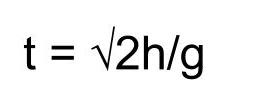# Projectile Motion Experiment

Instructor: Amanda Robb
In this experiment, you will learn how to create your own projectile motion experiment in order to calculate initial velocity of a horizontally launched projectile and the distance traveled.

## Introduction

 Goal: To calculate the initial velocity of a horizontally launched projectile and the distance traveled. Age: Middle school and up. Students must have some basic algebra skills and knowledge of physics. Safety concerns: Never launch a projectile at any living thing. Even something small like a marble ball can cause injury. Time to complete: Two hours

After studying for your physics exam for hours, you're pretty tired. As you reach to grab your notebook you bump your phone. It goes sliding across the table and off of the edge. Where should you reach to grab it before it hits the floor to stop the screen from shattering? This heart pounding scenario is one example of horizontal projectile motion. In horizontal projectile motion, an object is launched along a curved path in the horizontal direction. For a review of projectile motion you can watch this lesson: Projectile Motion: Definition and Examples.

Today, we're going to use two projectile motion equations to calculate the inital velocity of the ball as it leaves the table and the distance it will travel to the floor. For more information on the equations we'll be using you can look at this lesson: Uniformly-Accelerated Motion and the Big Five Kinematics Equations.

## Materials

• Marble
• Paper towel tube
• Tape
• Five books
• Small book
• Table
• Scissors
• Timer
• Ruler
• Notebook and pencil

## Steps

1. First, construct your ramp. Cut the paper towel tube in half to create a track.

2. Stack the books about 3 feet from the edge of a table. Attach the ramp to the book stack using tape.4. Now, measure the distance from the bottom of the ramp to the end of the table. Record this in your notebook.

5. Next, place an object like a smaller book at the end of the track to prevent the marble from flying off of the track, or have a friend catch it.

6. Get your timer ready and place your marble at the top of the ramp. Measure the time it takes to get from the bottom of the ramp to the end of the track. Record this value in your notebook.

7. Repeat step 6 three times and take an average to get a more accurate value for time.

8. Now, calculate the initial velocity by dividing distance by the average time:

v = d/t

9. Now that we know initial velocity, its possible to predict how long it will take the ball to hit the floor.

10. Measure the distance from the table to the floor and record this in your notebook as the height of the marble.

11. Next we will calculate where the marble should hit the floor. But, first we need to calculate time.

Since the marble is falling to the floor, the acceleration is equal to gravity. We can use the equation:12. Now that we know how long it will take the marble to hit the floor we can use our initial velocity to calculate how far it will go: (x)

x = v * t

To unlock this lesson you must be a Study.com Member.

### Register to view this lesson

Are you a student or a teacher?

### Unlock Your Education

#### See for yourself why 30 million people use Study.com

##### Become a Study.com member and start learning now.
Back
What teachers are saying about Study.com

### Earning College Credit

Did you know… We have over 160 college courses that prepare you to earn credit by exam that is accepted by over 1,500 colleges and universities. You can test out of the first two years of college and save thousands off your degree. Anyone can earn credit-by-exam regardless of age or education level.